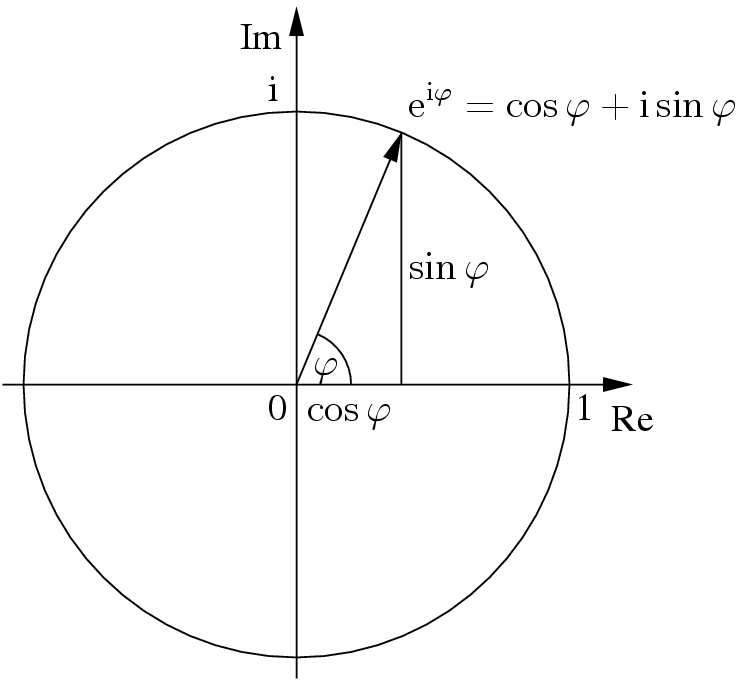On quite many online places I go by the handle cosinepi. I have it with Gmail, Facebook, Twitter, GitHub, Pinterest, LinkedIn, Instagram, and if you read Chinese, Renren and Weibo too.

I feel absolutely normal about this, until one day one of my friends asked me what cosinepi actually means. I feel it’s better to answer this, since I also find people having trouble pronouncing cosinepi.

In fact, cosinepi should be read as cosine-pi, meaning the trigonometric function cos applied to the constant pi, i.e., $$\cos(\pi)$$. It is a mathematical expression, namely, well quite obviously, the cosine of $$\pi$$, the ratio of a circle’s circumference to its diameter. The value of this express is simply minus one.

So the real question is, why do I choose this ID as my internet handle? There are three main reasons.

# cosinepi Means Great Ranking

I used to compete in the National Olympiad in Informatics and other national competitions in math, physics, chemistry, and English while I was in high school. That was a time when people were crazy about rankings. Specially, they were not satisfied with ranks in third, second, or first places, so they created something that literally translated to “special award” or “ultra special award”. So I thought to myself: why not extending the positive integers (as ordinals) to zero and negatives, such as zeroth place and minus-one-th place? You would save a bunch of additional words to describe what you’ve achieved, wouldn’t you? That’s why I decided to choose a handle that equals to the number minus one.

# cosinepi Is Mathematically Beautiful

If you are familiar with elementary school math (in China) you would know that the Euler’s identity $$e^{i\varphi} = \cos \varphi + i\sin \varphi$$ has been regarded the most beautiful mathematical equation. The special case, when $$\varphi = \pi$$, simplifies to $$e^{i\pi} = \cos \pi$$. When blended with the key sauce that $$\cos \pi = -1$$, this translates to $$e^{i \pi} + 1 = 0$$, which includes the five most important numbers in mathematics: 0, 1, $$i$$, $$e$$, and $$\pi$$. I simply cannot love this equation more. The host name for my site, Pi-Identity, also comes from this root.

# Handles Are Hard To Change

Once I’ve settled with the handle cosinepi, it is really a challenge for me to change. I have associated almost my everything with this handle, changing an Internet handle seems like changing me to another person. I currently do not see a need for such a change, and probably will never will. After all, what else can compete with the eternal beauty of Euler’s identity?